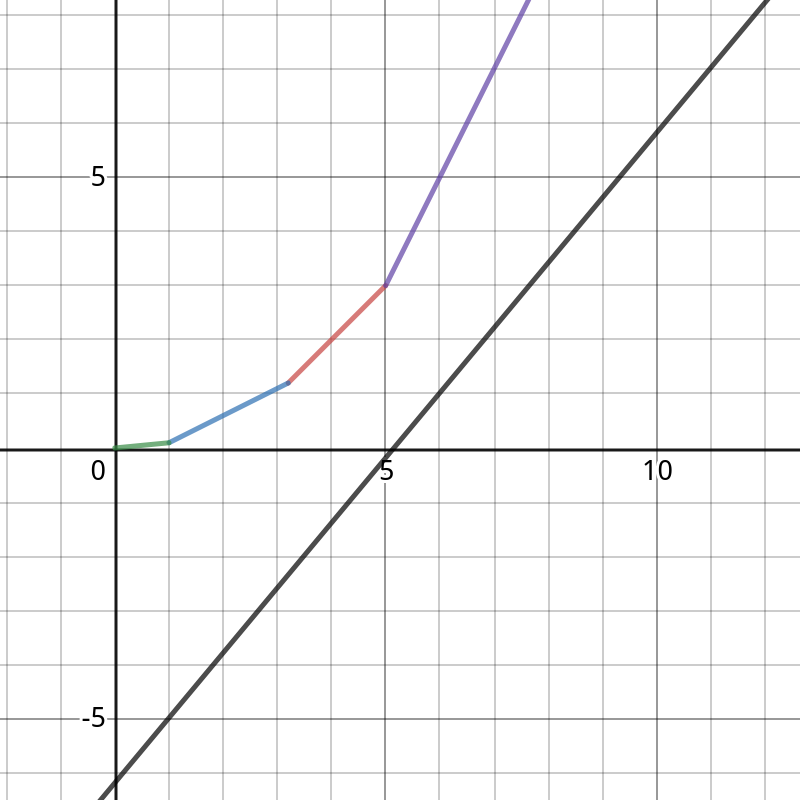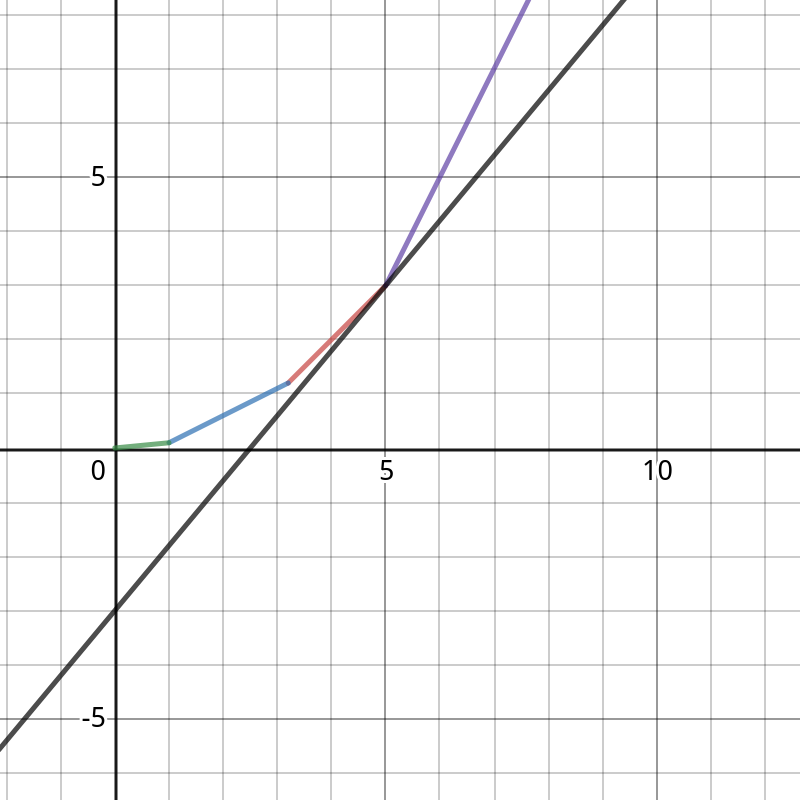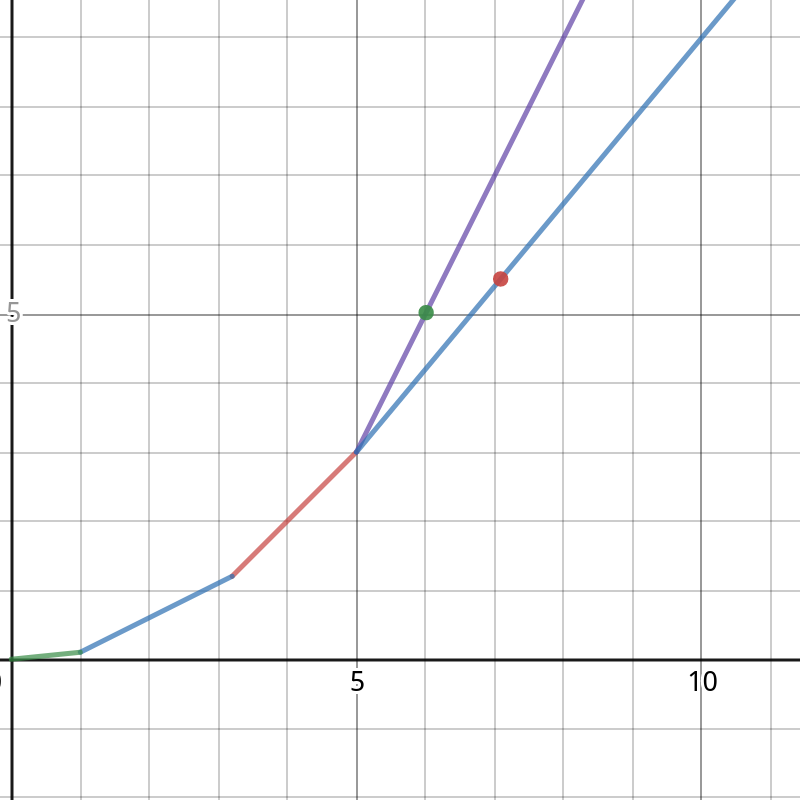# categories/刷题记录/共646篇文章zc
2019-12-21 19:47:00

f_i表示前i件玩具的制作费用

 f_i=\min_{j=1}^{i-1}(f[j]+(i-j-1+s_i-s_j-L)^2) 

a_i=s_i+i,b[i]=s_i+i+L+1

(都只与i有关)

x=b_j,y=f_j+{b_j}^2  y=2a_ix+(f_i-{a_i}^2) 

f_i含义转化为当上述直线经过点(x,y)(b_j,f_j+{b_j}^2)时,直线在y轴的截距上加上{a_i}^2

https://www.desmos.com/calculator/mqsdvzuaee1. while(s(q_h,q_{h+1})<2a_i)++h;
2. 当前队头的点最优,用这个点计算出f_i
3. while(s(q_{t-1},q_t)>s(q_{t-1},i))--t;
4. 队尾插入点izc
2019-12-21 19:47:00

# 玄学的最短路优化

ban:(禁用)

d(x,y)xy的最短距离zc
2019-12-21 19:47:00

[l,r]之间最小值位置为p,原数组为a

pre_ii前第一个比a[i]小的数的位置(用单调栈筛出)

f[l][r]表示以r为右端点,左端点在[l,r]的区间的答案

[l,p-1]求法类似zc
2019-12-21 19:47:00

 d(ij) = \sum_{x|i} \sum_{y|j}[gcd(x,y) = 1] 

n<m

 \sum_{i=1}^n \sum_{j=1}^m d(ij) \\ =\sum_{i=1}^n \sum_{j=1}^m \sum_{x|i} \sum_{y|j}[gcd(x,y) = 1] \\ =\sum\limits_{x=1}^n\sum\limits_{y=1}^m \left\lfloor\frac{n}{x}\right\rfloor \left\lfloor\frac{m}{y}\right\rfloor [\gcd(x,y)=1] 

x、y换成i、j

 \sum\limits_{i=1}^n\sum\limits_{j=1}^m \left\lfloor\frac{n}{i}\right\rfloor \left\lfloor\frac{m}{j}\right\rfloor[\gcd(i,j)=1] \\ =\sum\limits_{i=1}^n\sum\limits_{j=1}^m \left\lfloor\frac{n}{i}\right\rfloor \left\lfloor\frac{m}{j}\right\rfloor \sum_{k|gcd(i,j)}\mu(k) 

g(i)=\sum_{i=1}^n \left \lfloor \frac ni \right \rfloor

 ans=\sum_{k=1}^n\mu(k) g(\left \lfloor \frac nk \right \rfloor) g(\left \lfloor \frac mk \right \rfloor) \\zc
2019-12-21 19:47:00zc
2019-12-21 19:47:00zc
2019-12-21 19:47:00

https://www.luogu.org/blog/CSGO/solution-p3413zc
2019-12-21 19:47:00zc
2019-12-21 19:47:00zc
2019-12-21 19:47:00

\sum_{i=l}^{r}(f(i)-k) \le kd

Search
search